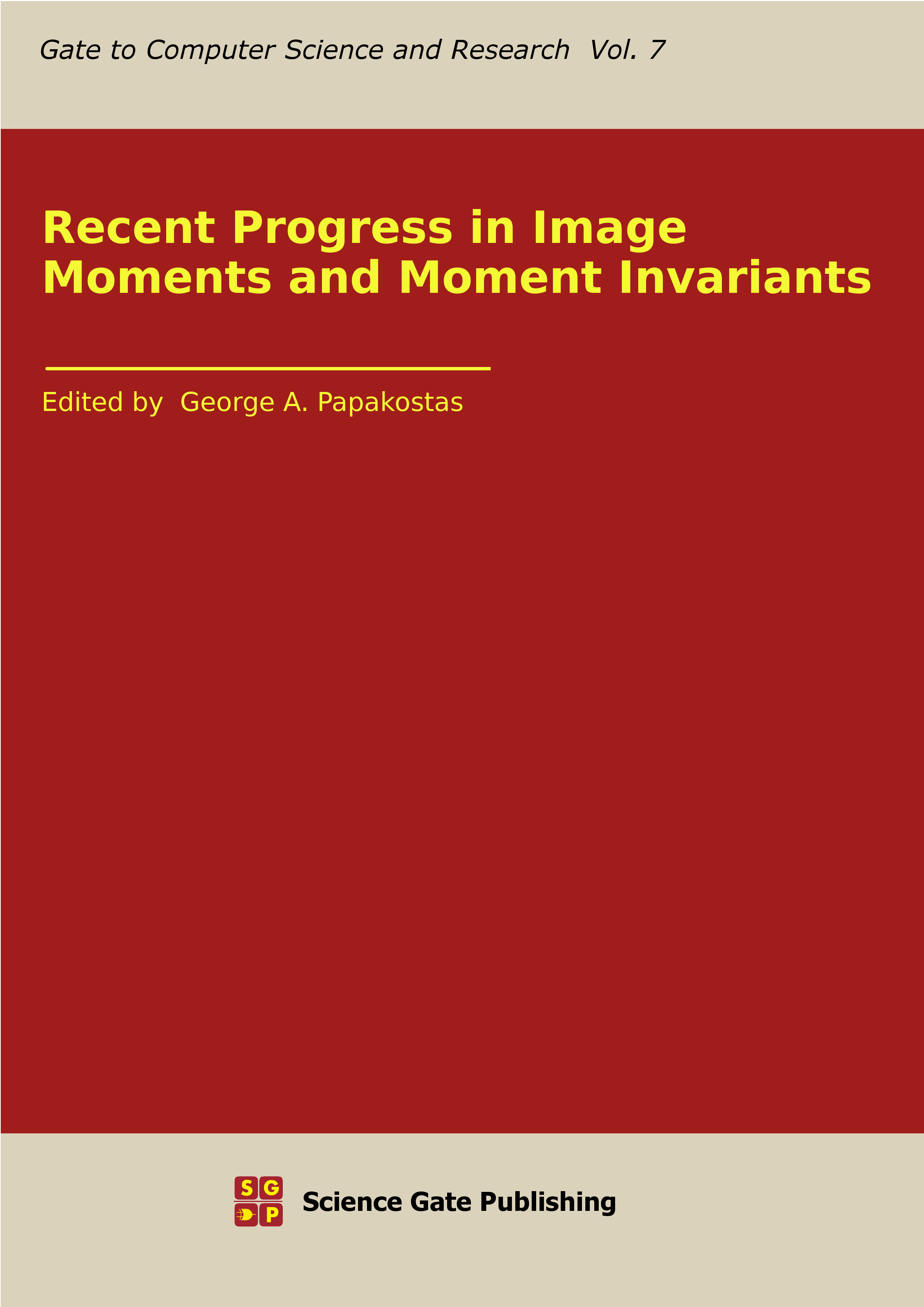# Recent Progress in Image Moments and Moment Invariants## Recent Progress in Image Moments and Moment Invariants

### GCSR Volume 7

Edited by

George A. Papakostas

(pages i-v)

#### Chapter 1 – Moment Invariants of Vector and Tensor Fields

Abstract

Vector and tensor fields are multidimensional data, where in each pixel/voxel the field is assigned to a vector or a matrix. The fields describe particle velocity, optical flow, stress and conductivity tensors, and similar phenomena. One of the challenging tasks is the invariant detection of patterns of interest. Invariants to total rotation and affine transformation of the field are desirable to accomplish this task. In this chapter, we review a recent development of this research area and show several practical applications on real data.

#### Chapter 2 – Radial Moments Based Object Recovery Under Symmetry Constraints

Abstract

In this chapter we develop a class of moment based methods for invariant image reconstruction with the selected degree of symmetry. An image function is expressed in terms of radial Zernike moments due to their invariance properties to isometry transformations and the ability to uniquely represent the salient features of the image.
The regularized ridge regression estimation strategy under symmetry constraints for estimating Zernike moments is introduced. The proposed extended regularization problem allows us to enforce the bilateral symmetry in the reconstructed object. This is achieved by the proper choice of two regularization parameters controlling the level of reconstruction accuracy and the acceptable degree of symmetry. As a byproduct of our studies, we propose an algorithm for estimating an angle of the symmetry axis which in turn is used to determine the possible asymmetry present in the image. The established image recovery under the symmetry constraints model is tested in a number of experiments involving image reconstruction and symmetry estimation.

#### Chapter 3 – Computation of 2D and 3D High-order Discrete Orthogonal Moments

Abstract

This chapter is about eliminating numerical instability and the error of high-order orthogonal moments by reducing terms in existing recurrence relations and the Gram-Smith orthonormalization process. Besides, the simplification of the terms of the recurrence relations with respect to n of the most used kernels is analyzed, such as Tchebycheff polynomials, Hahn polynomials, Krawtchouk polynomials, Charlier polynomials, and Meixner polynomials. Also, to guarantee the effectiveness of the proposed method, reconstructions of both 3D objects and high-resolution images are presented. The results presented in this chapter will help you utilize moments for processing, recognition, and analysis on 8K Full HD images and 3D objects with large dimensions.

#### Chapter 4 – Hyperspectral Image Classification by Chebyshev Moment Multifractal Profiles

Abstract

This chapter introduces Chebyshev moment multifractal profiles (CMMPs) used for hyperspectral image (HSI) classification. It is shown that CMMPs are effective texture descriptors and can be obtained through Chebyshev moments (CMs) for Hölder exponent images. It is also demonstrated that HSI classification based on the use of the proposed CMMPs can include the following steps: extracting the first few principal components (PCs) of HSIs, calculation of CMMPs from the extracted PCs, stacking PC-values and CMMPs into high-dimensional feature vectors, classification of the formed feature vectors by some supervised classifier (e.g., support vector machine (SVM) classifier). In the last part of the book chapter, results of spectral-spatial classification of two test HSIs using the proposed CMMP features are presented and discussed. It has been experimentally established that HSI classification by spectral features and CMMPs exhibits better performance than competitive methods of spectral-spatial HSI classification. In particular, it is shown that CMMP-based classification can outperform deep learning methods that are widely used for HSI classification in recent years.

#### Chapter 5 – Image Analysis by Fractional-order Orthogonal Moments

Abstract

Continuous orthogonal moments and discrete orthogonal moments, as a category of typical moments, have been widely used in image analysis. However, the order of these moments is limited to an integer and their fractional versions have not been studied sufficiently in the literature. This chapter provides an overview of fractional order orthogonal moments (FrOM) and their applications in image analysis. A detailed study of these moments is provided, including the mathematical development, properties, and computational aspects of these descriptors. Applications have been studied to validate the performances of the presented FrOMs, such as 2D/3D image reconstruction, RST invariance, 2D/3D image classification and image watermarking. The theoretical analysis and the experimental results show the superiority and the efficiency of the FrOMs compared to the classical versions in terms of the results obtained in the adopted image processing applications.